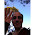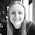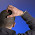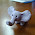## 20 December 2017

### New GCSE: Ratio

I've just marked my Year 11 mocks and noticed that ratio continues to be an area of difficulty. This isn't a surprise - we realised a few years ago that ratio questions on the new GCSE are much harder than they used to be. Here's an example of a straightforward ratio question - this is what ratio used to look like at GCSE:

The ratio of boys to girls at a school is 5:7
There are 600 children at the school.
How many boys are there at the school?

But GCSE questions are more challenging now. We're seeing a lot of 'ratio change' questions - this example, from Access Maths, was recently tweeted by Gayle Head (@maths_head):
How would you solve it? Here's an algebraic approach:
I've used equivalent fractions in my workings here - I think this approach really helps my students get the 5 and the 3 in the right place in the equation.

I've seen other teachers use totally different algebraic methods for questions like this (there's a nice example here).

I don't always use algebra for ratio problems - I also teach my students the 'scaling' method. This involves taking the initial ratio and finding a series of equivalent ratios until you find one that works (essentially finding x by trial and error). In this example, we start with 7:3 and multiply by two to get 14:6. Now if Alice started with 14 sweets and Olivia started with 6 sweets, then Alice giving three to Olivia would result in a ratio of 11:9, which is not what we want. So we try again. Our next equivalent ratio is 21:9, and again if we take three from Alice and give them to Olivia we'd get 18:12, which again is not equivalent to 5:3. So we keep going. Our next try is 28:12 which works.

Scaling is sometimes really time consuming. In this example we could have skipped straight to the correct answer if we'd realised that the total number of sweets has to split into 10 parts (ie 7:3) and also into 8 parts (ie 5:3). If we try the lowest common multiple of 10 and 8 then we get the answer really quickly.

We'd use the lowest common multiple if we were going to use bar modelling too.

On the subject of bar modelling - many of us have always drawn bars and boxes on the board when teaching ratio - bar modelling is most definitely not new! However, it is all the rage at the moment and increasingly used in both primaries and secondaries. Over the next few years we'll start seeing many Year 7s coming through to us using bar modelling as a standard approach for many problems, so it is sensible for all secondary teachers to at least know how it works.

If you're not familiar with bar modelling, Kris Boulton's TES article and William Emeny's blog post are a good place to start for the basics. Your local Maths Hub may run a bar modelling course. If you know the basics but struggle with the harder questions, check out this Twitter thread to see a bar model in action for a trickier ratio problem. It's also worth watching this excellent video on solving harder ratio problems using bar models from Colin Hegarty.

Bar modelling certainly is a clear and accessible approach for simple ratio questions. I would say though that for some trickier ratio questions it's not always quite as intuitive and obvious as expert bar modellers suggest it is.

Let's have a look at methods for another 'ratio change' question - this one is from Don Steward:
The algebra method I described above works very quickly. Using equivalent fractions and setting up an equation gives us 8(5x + 2) = 7(6x). The whole solution takes only a few lines of working.

Scaling works too:
5:6 gives 7:6 when Jan gains 2 marbles
10:12 gives 12:12 when Jan gains 2 marbles
15:18 gives 17:18 when Jan gains 2 marbles
20: 24 gives 22:24 when Jan gains 2 marbles
25: 30 gives 27:30 when Jan gains 2 marbles
30: 36 gives 32:36 when Jan gains 2 marbles
35: 42 gives 37:42 when Jan gains 2 marbles
40: 48 gives 42:48 when Jan gains 2 marbles - this simplifies to 7:8

Again, we can get there more quickly if we think about multiples. We start with a multiple of 11 and end with a multiple of 15, but we gained two marbles along the way. So we can list all multiples of 11 and all multiples of 15 and find a pair which are two apart.

11, 22, 33, 44, 55, 66, 77, 88
15, 30, 45, 60, 75, 90
So we started with 88 marbles.

Not all tricky ratio questions are in the form of these 'ratio change' problems. We also now get GCSE questions like this:

If the ratio a:b is 4:7, write a in terms of b

Though this may seem obvious to many of us (a is the smaller of the two, so a is four sevenths of b), writing the ratios as equivalent fractions can help students get their numbers the right way round (providing they are confident in rearranging equations).

Another type of question is this:

If the ratio a:b is 2:5 and the ratio b:c is 3:10, what is the ratio a:c?

Again, fractions might help.

A quicker alternative is scaling here. We can write a:b:c as one ratio if we get the b parts to match.

a: b can be written as 6:15
b:c can be written as 15:50
So a:b:c is 6:15:50
This shows that the ratio a:c is 6:50, which simplifies to 3:25

And here's another type of question:

Punch is made my mixing orange juice and cranberry juice in the ratio 7:2. Mark has 30 litres of orange juice and 8 litres of cranberry juice. What is the maximum amount of punch that Mark can make?

Again, I think that scaling is probably the quickest approach here. Multiplying the punch ratio by 4 gives us 28:8. This is the most punch we can make because we're using all the cranberry juice. So in total we're making 36 litres of punch.

In summary, there are a variety of approaches for solving trickier ratio problems - most can be solved efficiently using algebra, scaling or bar modelling. If you're teaching this topic for the first time at GCSE it's worth spending some time looking at the various methods. I think our students will need a lot of practice of numerous different types of ratio question to prepare for their GCSE.

Resources
I've created a lesson to accompany this post - download it from TES here.

Here are some other resources that you might find helpful:
There are loads more ratio resources in my number resources library.

I hope this post will be helpful when you teach ratio at GCSE. Do tweet me to let me know what methods you use if I haven't mentioned them here.

#### 17 comments:

1.My ratio pages don't get much attention - not sure why since I think they're instructive and easy to use. They don't support the particular type of harder questions described in the post (but I'll look to add something along those lines), but they do help understanding the concept of a ratio and it's utility.

Manipulation of ratio quantities: http://thewessens.net/ClassroomApps/Main/ratios.html?topic=number&path=Main&id=7
Introduction to the ratio concept:
http://www.thewessens.net/blog/2015/03/19/ratios-the-super-fractions/
Bar model visualisation of ratios:
http://thewessens.net/ClassroomApps/Models/BarModels/visualfractionratio.html?topic=models&path=Models&id=17
Multiplicative word problems:
http://thewessens.net/ClassroomApps/Models/BarModels/multiplicationwordproblems.html?topic=models&path=Models&id=8

Ken

1.Fantastic! Thanks Ken.

2.Thanks so much for your blog on ratio question types. Although I've been a maths teacher/tutor for over 30 year, ratio has always been a bug bear for me. I could wing it with old style gcse because I learnt the types of solutions required, however I have been stressed on the new types. This blog has made me think through ratios and I am certainly a lot happier.
Bryan

1.Excellent, I'm so pleased it helps.

3.On your fractions approach, a quick trick is to realise that a/c = a/b x b/c. Makes it quite quick to work out (That is, if the students are good with cancelling down when multiplying).

However, what I find confuses students about writing ratios as fractions is that it confuses the part:part idea of a ratio with the part:whole idea of a fraction. Perhaps that's why it's somewhat counter-intuitive.

Also, final point is that ratios (fractions) and vectors is another application of harder ratio questions that often pops up on the new GCSE.

Thanks for the post,
Farah

1.Thanks for the comment!

4.This is a fabulous resource on work that is missing from the new GCSE texts that I have seen. Lovely challeging questions to make students think.

1.Thanks! Glad it's helpful.

5.I've been using equivalent ratios for these type of questions.
Find what doesn't change - the total number of sweets.
Write ratios as equivalent ratios where the parts that doesn't change are the same.
3:7 has 10 parts, 3:5 has 8 parts
LCM of 8 and 10 is 40
Ratios are 12:28 and 15:25
Number of sweets given is 3.

Also works for following question
Ratio of blue to red counters in a bag is 1:2, I add 12 blue counters and the ratio is 5:7. How many red counters are in the bag?
What doesn't change? Red counters
LCM of 2 and 7 is 14
Ratios are 7:14 and 10:14
3 parts are 12 counters, 1 part is 4 counters and 14 parts are 56 red counters.

Also Jill is 4 times older than Jack. In 14 years time the ratio of Jack's age to Jill is 5:7. How old is Jill now?
Ratios are 1:4 and 5:7.
What doesn't change? The difference between their ages
Find two equivalent ratios where difference between them is the same.
4 - 1 = 3 and 7 - 5 = 2
LCM of 2 and 3 is 6
Equivalent ratios are
2:8 and 15:21
13 parts = 26 years, 1 part is 2 years, Jill is 16.

1.This is the approach I use. I think it's logical.

2.Thank you! Yes, this is logical. Same approach as bar modelling (but without the visual).

3.Oops, my mistake, third example should be .....in 26 years time the ratio of their ages is 5:7 .....
I did try to represent these using bar modelling at first but struggled to find a model that was intuitive and actually helped with the question. I would be grateful if anyone has ideas on this.

4.Although some bar modelling experts would disagree, I don't think bar modelling is intuitive/helpful for harder ratio questions. Bar modelling is fantastic for easier ratio questions, but when the questions get more complicated it's often really hard to figure out how to draw the scenario - definitely not as easy as some people make out!

6.Thank you for the post. Brilliant as usual.

I actually did the sweets question in my class once. I simply said that Alice fraction of sweets changed from 7/10 to 5/8 when she gave the 3 sweets away. If we just subtract those fractions, the fraction remaining, 7/10 - 5/8 = 3/40. This means that Alice originally had 40 sweets.

1.Hadnt considered tis method but I love it

2.Thanks Stephen. I guess it makes sense, as the fraction lost is equivalent to the 3 sweets divided by the total.

3.Love this! Thanks for sharing.